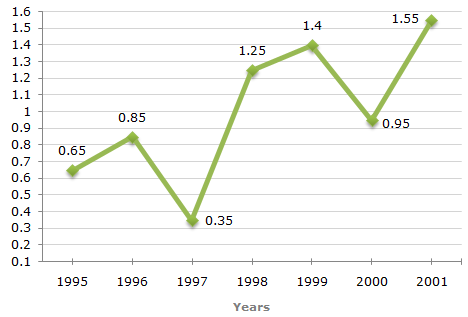# Data Interpretation - Line Charts - Discussion

Discussion Forum : Line Charts - Line Chart 2 (Q.No. 1)
Directions to Solve

The following line graph gives the ratio of the amounts of imports by a company to the amount of exports from that company over the period from 1995 to 2001.

Ratio of Value of Imports to Exports by a Company Over the Years.1.
If the imports in 1998 was Rs. 250 crores and the total exports in the years 1998 and 1999 together was Rs. 500 crores, then the imports in 1999 was ?
Rs. 250 crores
Rs. 300 crores
Rs. 357 crores
Rs. 420 crores
Explanation:

The ratio of imports to exports for the years 1998 and 1999 are 1.25 and 1.40 respectively.

Let the exports in the year 1998 = Rs. x crores.

Then, the exports in the year 1999 = Rs. (500 - x) crores.1.25 = 250x = 250 = 200       [Using ratio for 1998] x 1.25

Thus, the exports in the year 1999 = Rs. (500 - 200) crores = Rs. 300 crores.

Let the imports in the year 1999 = Rs. y crores.

 Then, 1.40 = yy = (300 x 1.40) = 420. 300Imports in the year 1999 = Rs. 420 crores.

Discussion:
12 comments Page 1 of 2.

Kushal said:   2 years ago
1998-I/E 125/100-5/4-250 cr/200 cr.

1999I/E 14/10 -2/5-420 cr/300 cr.

Ans: 420 cr.

Akber khan said:   4 years ago
(250/1.25)
= (250*100Ã·125),
= 25000Ã·125,
= 1000Ã·5,
= 200.
(1)

Tenzin Rabgay said:   4 years ago
How it comes 200? Can anyone explain?

Pavan shidarth said:   5 years ago
Thanks for your explanation @Devesh Sengar.

Francisco said:   6 years ago
Why did it go from division to multiplication?

Devesh sengar said:   7 years ago
Import/exp = 1.25 means = 125/100.

So, 250/exp (of 1998) = 125/100.
So exp = 200(of 1998).
So exp of 1999 = 500- 200 = 300.

Similarly,
imp(1999)/300 = 140/100.
imp = 420.
(3)

Arjun said:   7 years ago
Anyone knows the short tricks of this question?
(1)

Prakash said:   8 years ago
Can you explain the formulas used to find x and y in imports and exports?

Because I am not understanding 250/x and y/300 which formula is used here?

Prasanth said:   8 years ago
Can you explain the formulas used to find x and y in imports and exports?

RAJALAKSMI said:   8 years ago
Sir can you explain clearly?